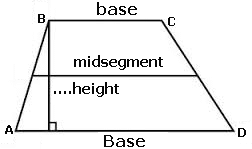### Trapezium

Trapezium in the USA or, trapezoid in the UK is a four-sided plane figure that has only one pair of-parallel straight line segments .
The sum of the internal angles, as well as the exterior angle is always equal to 360 degrees;
The non-parallel sides of the trapezium are called simply sides or legs, while, the two parallel straight line segments are called the bases. Every trapezoid has two bases
Read full text in Trapezium.
To calculate the elements of a trapezoid, enter data as requested. Use the point as a decimal separator. Ex 1,300.32 enter: 1300.32; The results will be shown after a click on Calculate.Trapezium Decimal places: Short base: Long base: Left side: Right Side: Area: Perimeter: Height: Midsegment: Diagonal A C: Diagonal B D: Angle A: Angle B: Angle C: Angle D: Note:

Note: The accuracy of the calculator and its applicability to particular cases is not guaranteed.